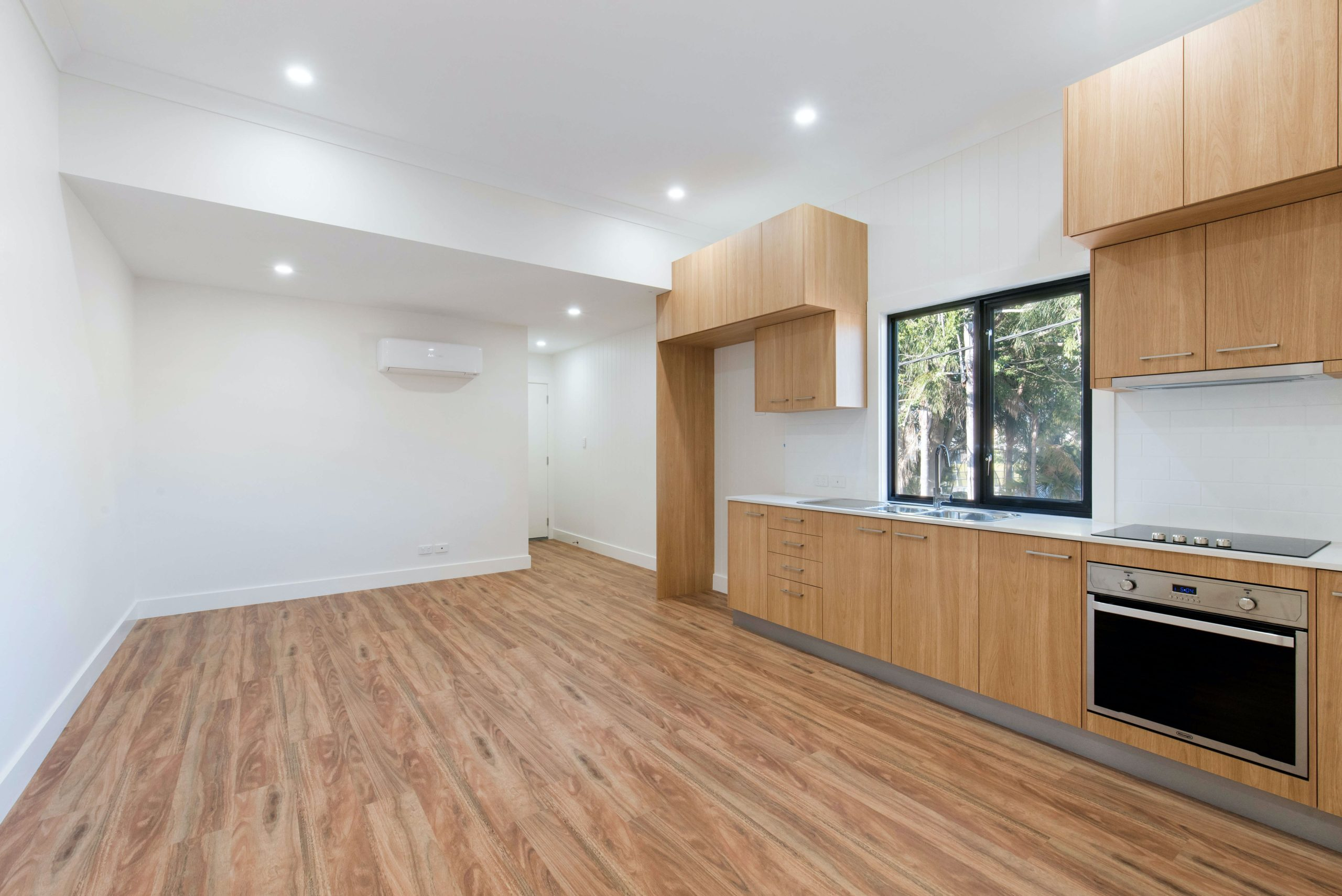# How To Calculate Your Flooring Plan Space

Redesigning your home is important for getting it to look the way you need it to, or, in order to be able to sell it at a premium to prospective buyers. Calculating the square footage of your flooring plan is vital to being able to ensure that your remodel goes right. For instance, if you need a new carpet, you have to know what your square footage is in order for you to get the right-sized carpet. Knowing your square footage is so important and here’s what you need to do to get the right square footage.

The Basics

Calculating your square footage is easy to do if you are dealing with rectangular spaces. All you have to do is multiply length by width. Although this is a basic principle, multiply length by width, it is an important principle to build upon.

The Real World

If you’ve ever visited the Polk Museum of Art at Florida Southern College, you will have seen examples of what we typically encounter in the real world. It’s a combination of rectangles and half circles. Here, we get to build on our basic principle. Finding the square footage of a place like that is very difficult. Here, what you want to do is section up your floor plan, so that you create rectangles, isolating the circles. You can then treat these areas as normal rectangles and then work on measuring the rest.

Calculating the square footage of the half-circlesis a little bit more difficult. You will need the help of a square footage calculator. Then, get the radius of the half-circle, which you do by measuring from the center of the half-circle, to its edges. Convert that number to feet by multiplying your number in inches by 0.0833333. Then, square the radius and multiply the result by pi (π) (around 3.141592). Divide the answer by half and you get the area of the half circles. These can then be added to the area of the rectangles. Then, you can invest in Full Coverage Painting & Flooring knowing that you have the right measurements.

Construction and Area Calculators

Using a construction calculator or area calculator is advisable if you want to speed up the process. You can even get an app to help you. These calculators will often allow you to input inches and feet. They make it easier to find the areas of odd shapes in flooring design. However, as we saw in our real world example, you can use basic mathematical principles to section off a floor plan to isolate complex parts of your floor plan, and then calculate the area of those complex plans on their own. This simplifies the problem, turning it from one big complex one, to something simpler.

These principles are appropriate regardless of the type of measuring standard you are working with, whether it’s yards, inches, feet, or centimeters. The principles are always the same. So don’t get bogged down trying to figure out how you will work with such-and-such a measurement. Stick to the principles.